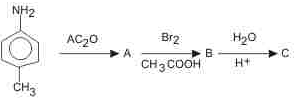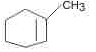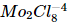Courses

# AFMC Chemistry Mock Test - 3

## 50 Questions MCQ Test AFMC Mock Test Series 2021 | AFMC Chemistry Mock Test - 3

Description
This mock test of AFMC Chemistry Mock Test - 3 for NEET helps you for every NEET entrance exam. This contains 50 Multiple Choice Questions for NEET AFMC Chemistry Mock Test - 3 (mcq) to study with solutions a complete question bank. The solved questions answers in this AFMC Chemistry Mock Test - 3 quiz give you a good mix of easy questions and tough questions. NEET students definitely take this AFMC Chemistry Mock Test - 3 exercise for a better result in the exam. You can find other AFMC Chemistry Mock Test - 3 extra questions, long questions & short questions for NEET on EduRev as well by searching above.
QUESTION: 1

Solution:
QUESTION: 2

Solution:
QUESTION: 3

### Which of the following has more unpaired d-electrons?

Solution:
QUESTION: 4

The hormone that helps in the conversion of glucose to glycogen is

Solution:
QUESTION: 5

Which salt can be produced by the reaction of carbon monoxide and caustic soda (NaOH)?

Solution:
QUESTION: 6

The quantum numbers of an atom can be defined on the basis of

Solution:
QUESTION: 7

α-D-Glucose and β-D-glucose differ from each other due to the difference in one of the carbons with respect to its

Solution:
QUESTION: 8The final product 'C' in the above reaction is

Solution:
QUESTION: 9

The structure of H₂O₂ is

Solution:
QUESTION: 10

The state of equilibrium refers to

Solution:
QUESTION: 11

The equilibrium constant in a reversible chemical reaction at a given temperature

Solution:
QUESTION: 12

Which of the following factors does not influence the rate of chemical reaction?

Solution:
QUESTION: 13

A foreign substance, that increases the rate of reaction, is called

Solution:
QUESTION: 14

Which of the following elements is/are not liquid at 30°C?

Solution:

Ga (melting point 29.8 ° C), Hg (melting point -38.8 ° C) and Cs (melting point 28.5 ° C) elements are liquids at room temperature, whereas Ge is a solid with melting point 937.4° C.

QUESTION: 15

Which pair of elements of atomic numbers given below will have similar chemical properties?

Solution:

In the periodic table, the elements belong to the same group have similar chemical properties. Atomic number-3 (i.e. Li ) and atomic number-11 (i.e. Na ) belong to the same group (i.e. alkali metals), thus they have similar chemical properties.

QUESTION: 16

For a process to be spontaneous

Solution:
QUESTION: 17

Which of the following ingredients is not used in sun burn creams?

Solution:
QUESTION: 18

Medicine which is an antibiotic is

Solution:
QUESTION: 19

cis and trans-2- betene are

Solution:
QUESTION: 20

Which of the following reactions actually take place?

Solution:
QUESTION: 21

The standard EMF of the cell Zn+Cu2⁺→Cu + Zn2⁺ is 1.10 V at 25°C. The EMF of the cell when 0.1 M Cu2⁺ and 0.1 M Zn2⁺ solutions are used will be

Solution:
QUESTION: 22

When CO₂ is bubbled through an aqueous solution of Na₂CO₃, the following is formed

Solution:
QUESTION: 23

Malachite is an ore of

Solution:
QUESTION: 24

Which of the following has the highest conductivity?

Solution:
QUESTION: 25

White enamel of our teeth is

Solution:
QUESTION: 26

Crude naphtha is a mixture of

Solution:
QUESTION: 27

on reductive ozonolysis yieldsSolution:
QUESTION: 28

A metal which does not liberate H₂ (g) from acids is

Solution:
QUESTION: 29

Which of the following on reaction with H₂S does not produce metallic sulphide ?

Solution:
QUESTION: 30

A radioactive element has a half-life of 20 mins. How much time should elapse before the element is reduced to 1/8th of the original mass ?

Solution:
QUESTION: 31

Loss of a β-particle is equivalent to

Solution:
QUESTION: 32

Ethyl bromide on treatment with alcoholic KOH gives

Solution:
QUESTION: 33

Which of the following is the true statement?

Solution:
QUESTION: 34

If N and S elements are present in organic compounds, then during Lassaigne's test both change into

Solution:
QUESTION: 35

Aniline when diazotised in cold and then treated with dimethyl aniline gives a coloured product. Its structure would be

Solution:
QUESTION: 36

Structure of ammonia is

Solution:
QUESTION: 37

Ammonium compound which on heating does not give NH₃ is

Solution:
QUESTION: 38

All the following decompose easily on heating to give oxygen except

Solution:

Manganese dioxide does not decompose on heating to give O2

QUESTION: 39

Which of the following statement is correct with respect to the property of elements with increase in atomic no. in the carbon family?

Solution:
QUESTION: 40

Bakelite is prepared by the reaction between

Solution:
QUESTION: 41

Which one of the following can be used as monomer in a polymerisation reaction?

Solution:
QUESTION: 42

The compound which could not act both as oxidising as well as reducing agent is

Solution:
QUESTION: 43

By increasing the temperature of a liquid, its vapour pressure

Solution:
QUESTION: 44

Which of the following liquid pair shows a positive deviation from Raoult's law?

Solution:
QUESTION: 45

Which of the following is hcp crystal structure?

Solution:
QUESTION: 46

Due to Frenkel defect, the density of ionic solids

Solution:
QUESTION: 47

Adsorption due to strong chemical forces is called

Solution:
QUESTION: 48

The first transition element is

Solution:
QUESTION: 49

In which one of the following transition metal complexes does the metal exhibit in zero oxidation state?

Solution:
QUESTION: 50

The oxidation state of Mo inion is

Solution: